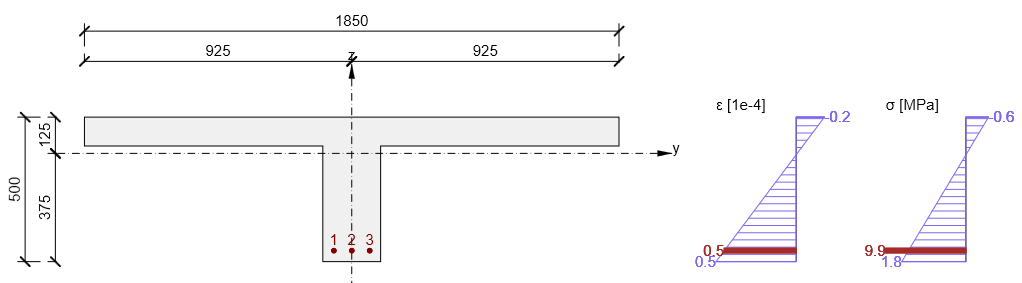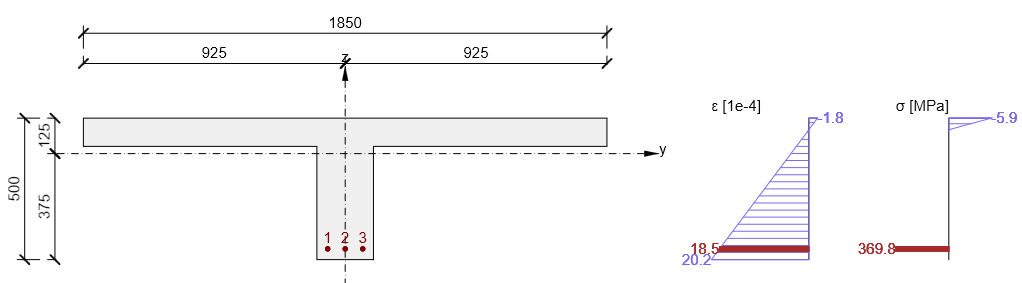# Calculation assumptions for SLS

$$The following assumptions are applied in the calculations according to caption 7.2 Stress limitation, 7.3.4 Crack width calculation, 7.4 Deflection control EN 1992-1-1Within the calculation the serviceability limit state we deals with two states that differ only in the tensile strength of concrete1. Uncracked cross-section the tensile strength of the concrete is not ignored. Concrete stress is directly proportional to the distance to neutral axis (linear stress distribution). Reinforcement stress is directly proportional to the distance to neutral axis (linear stress distribution). Concrete tensile stress is limited by value fct,eff according to art. 7.1 (2) EN 1992-1-1.$\textsf{\textit{\footnotesize{Uncracked section}}}$2. Fully cracked cross-section the tensile strength of the concrete is ignored. Concrete stress is directly proportional to the distance to the neutral axis (linear stress distribution). Reinforcement stress is directly proportional to the distance to the neutral axis (linear stress distribution).$\textsf{\textit{\footnotesize{Cracked section}}}$$$